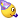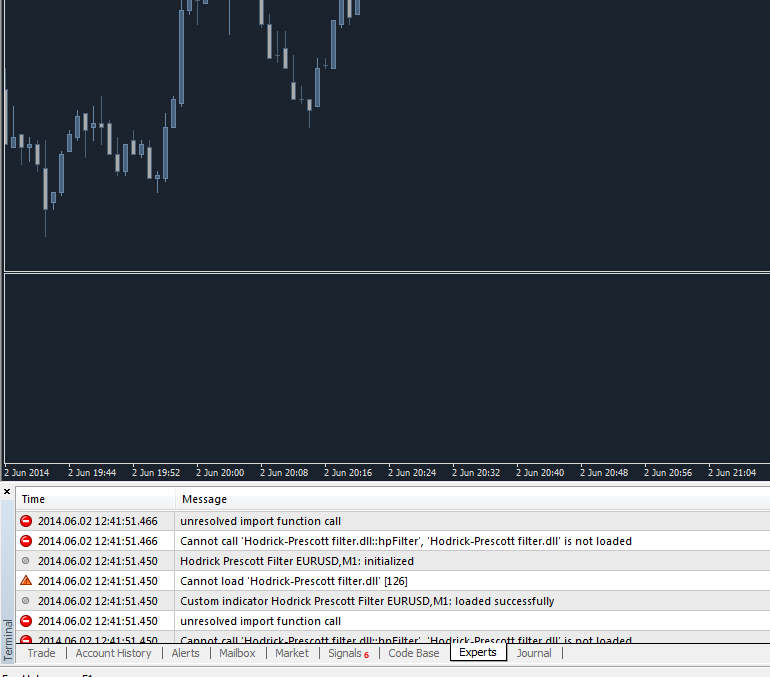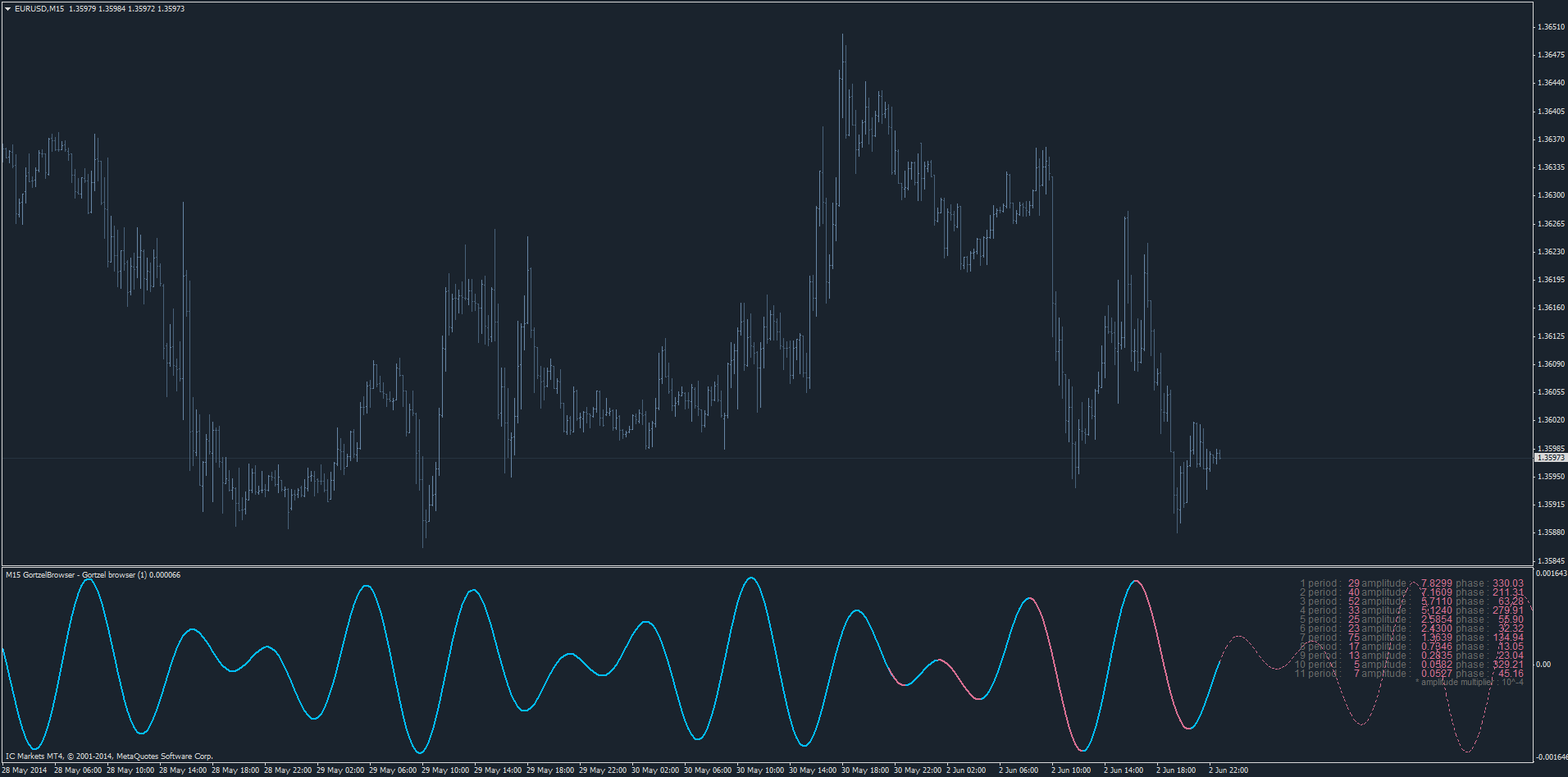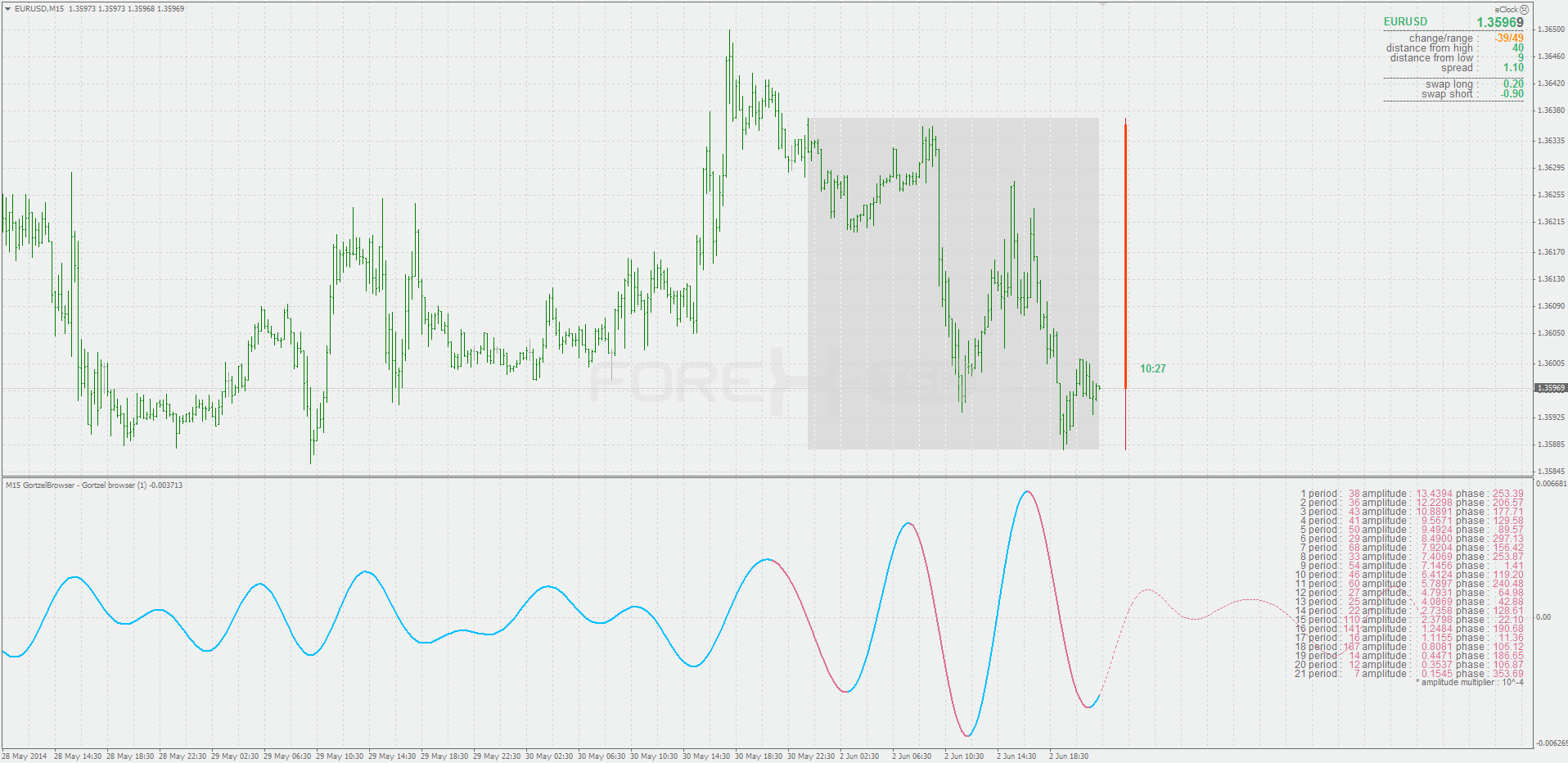# Requests & Ideas - page 324161

See the previous postDLL too please?Files:
dll.png 25 kb163389

DLL too please?Here you go : hodrick-prescott_filter.zip

Files:161

Here you go : hodrick-prescott_filter.zip

It's working! thanks.Files:
gb_1.gif 94 kb537

Please see the following tape histogram indicator. I would like for you to code it so that it allows me to have multiple periods, multiple timeframes and different obos for each period.

ccistudy2.01nrphistomtf.mq4

Example:

A. Period 50, obos 50 and -50 in 240 hrs.

B. Period 70, obos 100 and -100 in 1440 hrs.

Results:

1. If A above 50 and increasing and B above 100 and increasing = Aqua 2. If A below -50 and decreasing and B below -100 and decreasing = Yellow 3. If A above 50 and B above 100, but one increasing and one decreasing = Blue 4. If A below -50 and B below -100, but one decreasing and one increasing = Red 5. If A above 50 and B above 100, but both decreasing = Dark Blue 6. If A below -50 and B below -100, but both increasing = Maroon 7. If either A or B or both are not in obos zone = Grey

If buffers aren't enough for this to be coded, then code based on the following results:

1. If A above 50 and increasing and B above 100 and increasing = Aqua 2. If A below -50 and decreasing and B below -100 and decreasing = Yellow 3. If A above 50 and B above 100, but either A or B or both are decreasing = Blue 4. If A below -50 and B below -100, but A or B or both are increasing = Red 5. If either A or B or both are not in obos zone = Grey

Hope the above explanation is clear enough for you. Thank you in advance again Mladen.

Files:163389

rayphua:

Please see the following tape histogram indicator. I would like for you to code it so that it allows me to have multiple periods, multiple timeframes and different obos for each period.

ccistudy2.01nrphistomtf.mq4

Example:

A. Period 50, obos 50 and -50 in 240 hrs.

B. Period 70, obos 100 and -100 in 1440 hrs.

Results:

1. If A above 50 and increasing and B above 100 and increasing = Aqua 2. If A below -50 and decreasing and B below -100 and decreasing = Yellow 3. If A above 50 and B above 100, but one increasing and one decreasing = Blue 4. If A below -50 and B below -100, but one decreasing and one increasing = Red 5. If A above 50 and B above 100, but both decreasing = Dark Blue 6. If A below -50 and B below -100, but both increasing = Maroon 7. If either A or B or both are not in obos zone = Grey

If buffers aren't enough for this to be coded, then code based on the following results:

1. If A above 50 and increasing and B above 100 and increasing = Aqua 2. If A below -50 and decreasing and B below -100 and decreasing = Yellow 3. If A above 50 and B above 100, but either A or B or both are decreasing = Blue 4. If A below -50 and B below -100, but A or B or both are increasing = Red 5. If either A or B or both are not in obos zone = Grey

Hope the above explanation is clear enough for you. Thank you in advance again Mladen.

Just to clarify :

Combining different time frames into one state makes it recalculate (repaint) to the highest time frame used, so it is not possible to use it as signals (which makes alerts and arrows uselles in that version). Is that what you are looking for?537

Just to clarify : Combining different time frames into one state makes it recalculate (repaint) to the highest time frame used, so it is not possible to use it as signals. I s that what you are looking for?

Yes I understand that if I use multiple timeframes combined, the signals cannot be used. I want the flexibility in the indicator and I may not necessarily combine multiple timeframes but if I do I would like the indicator to allow for that. Thanks Mladen for looking into this.537

rayphua:
Yes I understand that if I use multiple timeframes combined, the signals cannot be used. I want the flexibility in the indicator and I may not necessarily combine multiple timeframes but if I do I would like the indicator to allow for that. Thanks Mladen for looking into this.

Hi Mladen, any progress with this? Thanks.163389

rayphua:
Hi Mladen, any progress with this? Thanks.

rayphua

Working on it537